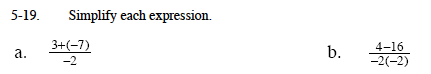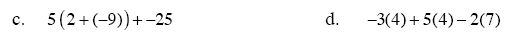### Home > MC1 > Chapter 5 > Lesson 5.1.2 > Problem5-19

5-19.Simplify the numerator first.

$\frac{3\;+\;(-7)}{-2}\;=\;\frac{-4}{-2}$

Next, divide the numerator by the denominator.

$\frac{-4}{-2}\;=\;2$

2

Follow the same steps as in part (a). Remember to simplify both the numerator and the denominator before dividing.Remember to work from within the parentheses outward.

−60

Make sure to compute each term before adding.